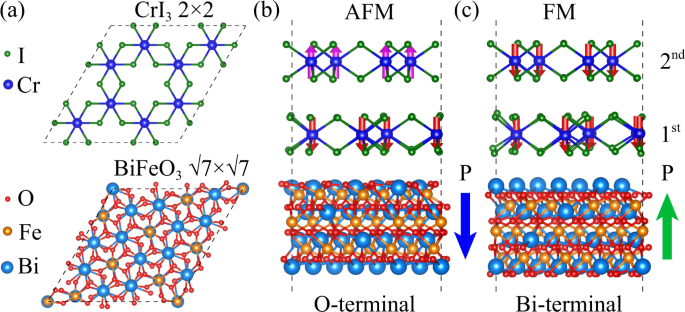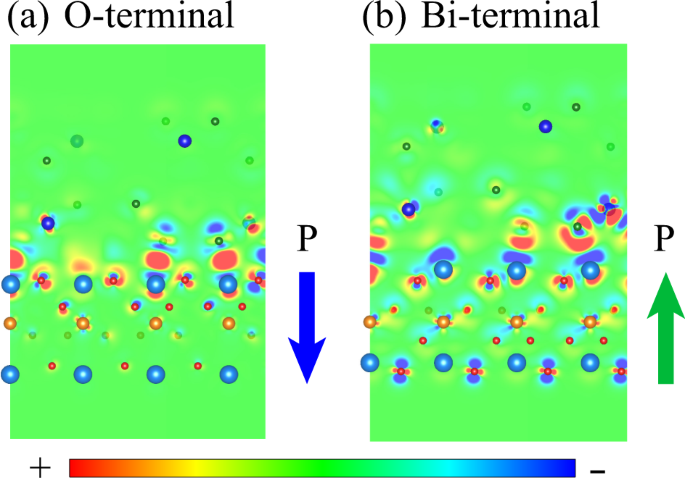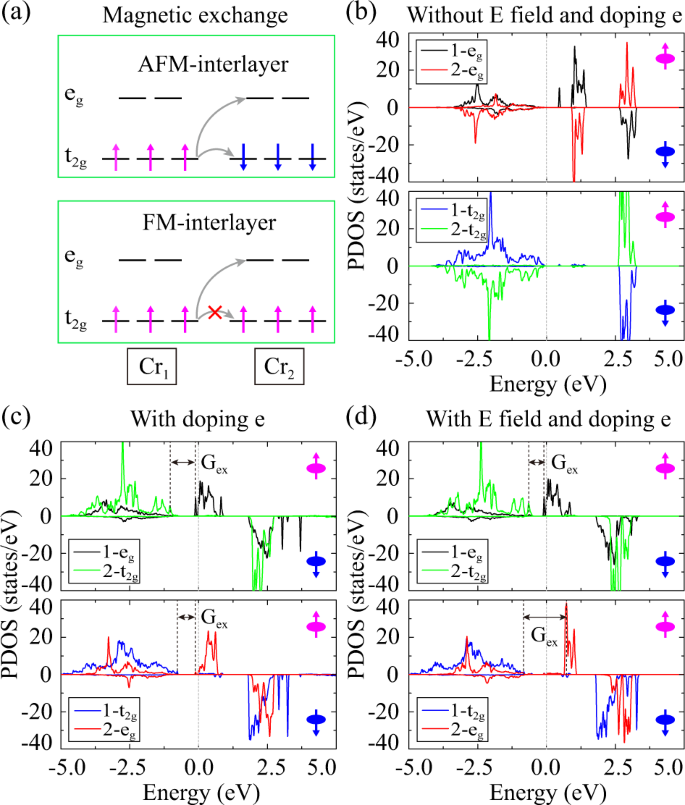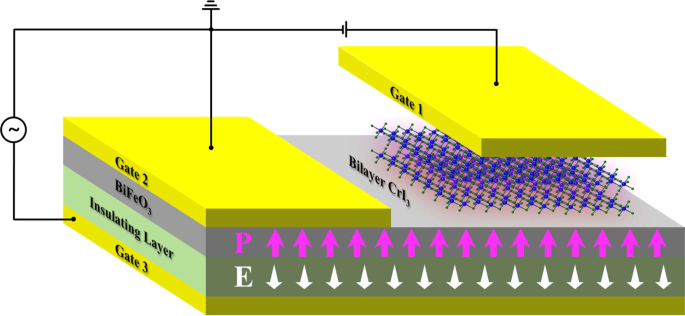## Introduction

In the past two decades, the multiferroic heterostructure composed of traditional magnetic material (such as iron, cobalt, and their alloys) and perovskite oxide ferroelectric (POF) has been widely studied1,2, due to its great potential in realizing large magnetoelectric coupling at room temperature. However, the large number of dangling bonds at the interface easily induces significant orbital hybridizations and even the ion migration between the magnetic material and the ferroelectric oxide (strong interface interaction)3,4. Such strong interface interaction usually leads to the irreversible destruction of the interface magnetic structure, and ultimately reduces the cycle life of the spintronic devices5,6,7,8,9.

Recently, the van de Waals (vdW) heterostructures engineering, via the stacking of layered systems with different properties, has provided a way to realize intriguing physical properties10,11,12,13,14. For example, group-IV monolayers15,16,17, 1T′ transition metal dichalcogenides18, and transition metal halides/oxides19,20,21,22, had been reported to be topological materials. Simultaneously, SnTe23, In2Se324, and CuInP2S625 had been experimentally demonstrated to be two-dimensional ferroelectric (2DFE) materials. Meanwhile, two-dimensional ferromagnetic (2DFM) materials, such as CrI326, Cr2Ge2Te327, Fe3GeTe228, and VSe229 had been successfully fabricated. Constructing heterostructures of 2DFE and 2DFM potentially provides a generally applicable route to create 2D multiferroics and magnetoelectronic couplings. Such heterostructure is expected to have vdW interface interaction due to the lack of dangling bonds, which is particular suitable for the infinite cycle life spintronic devices. While, a fundamental question is whether the vdW interlayer can induce the strong magnetoelectric coupling.

Up to now, several theoretical investigations have been performed to realize the magnetic phase transition (MPT) by manually changing the direction of dielectric polarization in the 2DFM/2DFE heterostructures, e.g., Cr2Ge2Te6/In2Se330, CrI3/Sc2CO231,32, and MnCl3/CuInP2S633. It had been verified that the magnetic ground state transition can be achieved even in the framework of vdW interface interaction30,31,32,33, because the electronic structure of the 2DFM is very sensitive to the charge transfer or electric field from the 2DFE31. However, a much larger external electric field is required to realize MPT in the 2DFM/2DFE heterostructure, due to the nature of weak ferroelectricity of 2DFE compared to POF materials.

Here by means of density functional theory (DFT) calculations (Methods are shown in Supplementary Materials), we propose a strategy for a small electric field controlling of MPT in the 2D magnets, i.e., 2DFM/POF heterostructures. We illustrate the feasibility of such strategy in the bilayer CrI3/BiFeO3(001) heterostructure, where BiFeO3 has much stronger electrical polarization to the 2DFM which can be flipped under a small electric field. Interestingly, we find the interface interaction between CrI3 and BiFeO3 is also vdW type, and the interlayer magnetic coupling of bilayer CrI3 can be efficiently switched between FM and antiferromagnetic (AFM) types by flipping the dielectric polarization. Additionally, we reveal the competing effect on the MPT between electron doping and the electric field induced by the BiFeO3.

## Results

### CrI3/BiFeO3 multiferroic heterostructures

We chose bilayer CrI3 with the monoclinic lattice and C2/m space group symmetry (the HT phase) as the 2DFM material34, which exhibits the FM intralayer and AFM interlayer magnetic exchange couplings that are reported by Xu’s group26. On the other hand, the R3c BiFeO3 is chosen as the POF material due to its room temperature reversible ferroelectricity and large electric polarization. The multiferroic heterostructure was constructed by stacking bilayer CrI3 on BiFeO3(001) surface. Considering that the optimized lattice constant of CrI3 is 7.00 Å and that of BiFeO3(001) is 5.64 Å, the 2 × 2 unit cell of CrI3 is commensurate to the $$\sqrt{7}\times \sqrt{7}$$ surface unit cell of BiFeO3(001) with a lattice mismatch of 6.59%, as shown in Fig. 1(a). We have considered three typical stacking configurations for CrI3 on O(Bi)-terminal of BiFeO3(001), i.e., the bottom CrI3 layer being on top, bridge, and hollow positions of the top O(Bi)-terminal layer (see Supplementary Fig. 1). After fully structural optimization, we find that the hollow (bridge) structure is the most stable for Bi-terminal (O-terminal) heterostructure (see Table 1).Fig. 1: The magnetic phase transition was induced by polarization reversal.

To quantify the interaction between CrI3 and BiFeO3(001), we calculated the binding energy Eb35, defined as Eb = (ECrI3 + EBiFeO3Etot)/NI. Here Etot, ECrI3, and EBiFeO3 are the total energies of the CrI3/BiFeO3(001), the bilayer CrI3, and the clean BiFeO3(001) surface, respectively. NI is the number of I atoms at the interface. The calculated Eb are shown in Table 1. For all the structures, the binding energies (0.34–0.51 eV I−1) are comparable to that of CrI3 on semiconductor substrate (0.28–0.41 eV Cr−1), implying the nature of vdW interaction between CrI3 and BiFeO314. Moreover, the average Bi–I (O–I) bond lengths at the interface are in the range of 3.75–3.79 (3.18–3.31) Åfor Bi-terminal (O-terminal). Considering that the atomic radii of I, O and Bi atoms are 1.40 Å, 0.66 Å, and 1.56 Å, respectively, this result confirms the non-bonded interface interactions. We have additionally calculated charge density distributions of the CrI3/BiFeO3(001) heterostructure (Supplementary Fig. 2) and found there is little charge density overlap at the interface. Therefore, one can conclude that the interface interaction between CrI3 and BiFeO3 is vdW type, where the migration of ions from BiFeO3 to CrI3 during the ferroelectric polarization inversion hardly occurs. Such multiferroic heterostructure is expected to be free of the flipping cycle-life issue.

### Tunable interlayer coupling of bilayer CrI3 and mechanism for interfacial multiferroicity

The total energy calculations further show that BiFeO3(001) can significantly affect the magnetic interlayer coupling of bilayer CrI3. As shown in Fig. 1, both the FM and AFM interlayer magnetic configurations of bilayer CrI3 had been considered. It is found that the total energies of their obviously depend on the dielectric polarized directions of BiFeO3(001), i.e., P↓ polarization when the O atoms move up along  direction (O-terminal, Fig. 1b), and P polarization on the contrary (Bi-terminal, Fig. 1c). As shown in Table 1, among all the stacking configurations (bridge/hollow/top) the FM (AFM) interlayer-coupling phase of bilayer CrI3 always has the lowest total energy when BiFeO3(001) is in the P↑ (P↓) state. This feature shows that the magnetic phase of bilayer CrI3 can be well switched by flipping the dielectric polarized directions of BiFeO3(001), which is easily realized under a small electric field. Note that the energy differences ΔE between FM and AFM states in both the P↑ (P↓) states are in range of 9.2–20.6 meV. The distinguish energy differences of FM and AFM states with ferroelectric polarization flipping indicate that the system has a strong magnetoelectric coupling.

It is desirable to understand the origin of MPT from AFM to FM for CrI3 on BiFeO3(001). In general, three combined factors induced by the BiFeO3 substrate can be responsible to the MPT: (1) structure reconstruction (deformation) of CrI3 induced by BiFeO3, (2) charge transfer effect from BiFeO3, and (3) electric field (electric polarization) effect from BiFeO3. In the following, we will distinguish the roles of these factors on the MPT via studying the bilayer CrI3 detached from BiFeO3. The DFT calculations show that the vdW interaction from BiFeO3 gives rise to the structure reconstruction of bottom CrI3 layer to a certain extent (see Fig. 1c). In order to clarify the influence of structure reconstruction to interlayer magnetic coupling, we calculated the total energy differences between FM and AFM phases of bilayer CrI3 detached from BiFeO3(001). As shown in Table 2, the energy differences (Δ E = EFMEAFM) of all the CrI3 in different configurations are positive. This feature means that the MPT in bilayer CrI3 is not attributed to the structure reconstruction. In addition, we have calculated the most stable phase of unrelaxed bilayer CrI3 structures, and found it has the same magnetic ground state, i.e., AFM state, as that of the relaxed one (see Supplementary Table 1). This result confirms that the geometry distortion of bilayer CrI3 induced by surface as well as other effects do not change the magnetic ground state.

Then, we explored the charge transfer effect on the MPT of bilayer CrI3. Figure 2a, b shows the calculated differential charge density distributions of CrI3/BiFeO3 heterostructure, where obviously charge transfer from CrI3 (BiFeO3) to BiFeO3 (CrI3) appears for P↓ (P) polarization. We have additionally estimated the amount of transferred charge and found it is sizable in both cases, i.e., 0.48 e per supercell from CrI3 to BiFeO3 for P and 1.63 e per supercell from BiFeO3 to CrI3 for P↑, respectively. In order to understand the mechanism of the charge transfer, we calculated the density of states (DOS) by using the Heyd–Scuseria–Ernzerhof (HSE) method sketched in Supplementary Fig. 3. For the O-terminal case, there are unsaturated O atoms in the top layer of BiFeO3 at the interface, which makes the surface state of BiFeO3 becomes metallic [see Supplementary Figure 3(b)]. Therefore, the charge transfer at the interface is mainly determined by the electronegativity of the interface atoms. The electronegativity of O (3.44) atom is larger than that of I (2.66) atom, which leads to the 0.48 e per supercell transferred from CrI3 to BiFeO3(001). For the Bi-terminal case, on the other hand, the O atoms on the top layer are saturated by Bi atoms (see Fig. 1), which induces the band gap for the interface state and thus allows the HSE investigations. As shown in Supplementary Figure 3(f), the valence-band maximum of BiFeO3(001) overlaps with the conduction-band minimum (CBM) of CrI3, which corresponds to a type III band alignment. Such phenomenon is attributed to the electron transfer from BiFeO3 to CrI3, which results in the upshift (downshift) of CBM energy level of BiFeO3(CrI3) in the heterostructure in comparation with the isolated ones [see Supplementary Fig. 3(d–f)].Fig. 2: 2D charge density difference of CrI3/BiFeO3(001) heterostructure in hollow configuration.

To further clarify the mechanism of electron doping on MPT of bilayer CrI3, we have systematically calculated the electron doping concentration (n) dependence of total energy differences between FM and AFM phases (ΔE) for bilayer CrI3 detached from Bi-terminal BiFeO3(001) with hollow configuration. Here we simulated the electron-doping effect through doping H atom in the hollow position of the first CrI3 (see Supplementary Fig. 4). As shown in Fig. 3a, ΔE monotonically decreases with doping concentration increases, where the MPT from AFM to FM appears when n > 0.5 e per supercell. This value is much smaller than that transferred from BiFeO3 to CrI3 (1.63 e per supercell), thus the charge transfer is expected to be a dominating factor to the MPT of bilayer CrI3.Fig. 3: Electric field and electron doping tune the magnetic phase transition of bilayer CrI3.

In addition, we have explored the effect of electric field on MPT of bilayer CrI3, which is originated from the P↑ polarization of BiFeO3(001). As shown in Fig. 3b, ΔE of detached CrI3 monotonically decreases with the increase of electric field, where the MPT occurs when the electric field becomes larger than 0.9 V Å−1. On the other hand, the equivalent electric field from BiFeO3(001) is estimated to be about 0.15 V Å−1. This result shows that the electric field from BiFeO3 has minor effect on the MPT of bilayer CrI3.

To further clarify the combined effect of charge transfer and the additional electric field on the MPT of bilayer CrI3, we have investigated the total energy difference Δ E of bilayer CrI3 detached from BiFeO3(001) in three cases, i.e., Case 1 without electric field and electron doping (only structure reconstruction effect is considered), Case 2 with only electron doping (1.5 e), and Case 3 with electron doping (1.5 e) and the additional electric field (0.5 V Å−1). As shown in Table 3, ΔE significantly decreases from 18.40 meV (Case 1) to −54.09 meV (Case 2) with only electron doping, whereas it increases to −3.63 meV (Case 3) with the additional electric field 0.5 V Å−1. This result shows that the combined effect of electron doping and electric field on the MPT of bilayer CrI3 is inferior to that of the electron doping alone, although the electric field alone also benefits to the MPT from AFM to FM phase. This feature shows that the electric field has an effect of weakening the FM phase in an electron-doping environment.

To reveal the underlying mechanism of charge transfer and electric field on the MPT of bilayer CrI3, we explored the interlayer exchange interactions between Cr atoms with both FM and AFM spin configurations. As indicated in Fig. 4a, the hopping between t2g and t2g orbitals is allowed for the AFM spin configuration but prohibited for the FM spin configuration according to the Pauli exclusion principle. This feature means that the t2gt2g (t2geg) orbital hybridizations give rise to the AFM (FM) phase of bilayer CrI3 from the viewpoint of the Hund’s coupling36,37. Figure 4(b–d) further shows the calculated the projected density of states (PDOS) of bilayer CrI3 in Cases 1–3 discussed above, where the 1-eg and 1-t2g (2-eg and 2-t2g) represent first layer (second layer) Cr eg and t2g orbitals, respectively. As one can see from Fig. 4b, the interlayer t2g-t2g orbitals have strong hybridization without electron doping and electric field (Case 1), in agreement with the AFM spin-exchange model in the upper panel of Fig. 4a. When only electron doping is applied to bilayer CrI3 (Case 2), the t2gt2g orbital hybridization becomes much weaker, whereas the t2geg hybridization becomes obviously stronger due to the downshift of eg energy levels (the exchange interaction strength between eg and t2g orbitals is inversely proportional to the virtual exchange gap (Gex), Fig. 4c). This characteristic is consistent with the FM spin-exchange model in the under panel of Fig. 4a. When the electric field is additionally applied to the electron-doped CrI3 (Case 3), Gex significantly increases (under panel of Fig. 4d), indicating that the t2geg orbitals hybridization becomes weaker. This feature explains why the electric field in the electron doping environment can weaken the FM phase discussed above.Fig. 4: Interlayer spin-exchange model and calculated PDOS for bilayer CrI3 detached from Bi-terminal BiFeO3(001) (hollow configuration).

To quantitatively investigate the effects of electron doping and the additional electric field on the interlayer exchange coupling of bilayer CrI3, we constructed a Heisenberg model for the bilayer CrI3 detached from Bi-terminal BiFeO3(001) (hollow configuration) with the Hamiltonian as

$$H={E}_{0}+{\sum }_{i,j}{J}_{1\parallel }{S}_{i}\cdot {S}_{j}+{\sum }_{k,l}{J}_{2\parallel }{S}_{k}\cdot {S}_{l}+{\sum }_{i,k}{J}_{\perp }{S}_{i}\cdot {S}_{k},$$
(1)

where E0 is the ground state energy independent of the spin configurations. Si, Sj, Sk, and Sl represent the magnetic moments at sites i, j, k, and l, respectively. J and J denote the intralayer and interlayer exchange interactions, respectively. We adopted J1 and J2 to denote the nearest-neighbor intralayer Cr-Cr exchange interaction of the first and second CrI3 layer, respectively. J1 and J2 are the nearest-neighbor and second-neighbor interlayer Cr-Cr exchange interactions [Supplementary Fig. 5(a)], respectively. The details for the calculation of exchange parameters based on the above Hamiltonian and total energy calculations using DFT are shown in the Supplementary material (see Supplementary Fig. 5 and Supplementary Table 2).

The obtained results for Cases 1–3 are summarized in Table 3. As one can seen, in all cases J1 > 0 (contributes to AFM phase) and J2 < 0 (contributes to FM phase). This feature shows that J1 is dominated by exchange interaction between Cr half-filled t2g orbitals which induces an AFM coupling, while J2 is dominated by a virtual excitation between the Cr half-filled t2g orbitals and the empty eg orbitals which leads to a FM coupling. Therefore, the ground state of bilayer CrI3 is determined by the competition between nearest-neighbor and second-neighbor interlayer Cr–Cr exchange interactions. Considering that the number of nearest-neighbor and second-neighbor interlayer Cr–Cr interaction pairs are the same all the three cases, the MPT of CrI3 can be predicted by the value of $${\overline{J}}_{{{{\rm{\perp }}}}}$$ = J1 + J2. In case without electric field and electron doping (Case 1), $${\overline{J}}_{{{{\rm{\perp }}}}}$$ = 0.26 meV, indicating the AFM ground state from the Heisenberg model which agrees well with the direct DFT calculations38. When the electron doping is applied (Case 2), the J1 decreases little whereas J2 nearly has a double enhancement (-1.09 to -2.08 meV), leading to a strong FM ground state ($${\overline{J}}_{{{{\rm{\perp }}}}}$$ = −0.75 meV). Additionally, when both the electron doping and the electric field are applied (Case 3), J2 significantly changes from −2.08 to −1.22 meV but J1 only decreases a little, resulting a weak FM ground state ($${\overline{J}}_{{{{\rm{\perp }}}}}$$ = −0.05 meV). These results reveal that electron doping and the additional applied electric field have significant but opposite influence on the second-neighbor interlayer Cr–Cr exchange interaction, the variation of which is the origin of MPT in bilayer CrI3. We have also calculated the exchange parameters in the three cases for the bilayer CrI3 without geometry reconstruction, and obtained the similar results (see Supplementary Table 3 and 4), which confirms the intrinsic nature of competition between electron doping and the additional electric field on the interlayer exchange coupling of bilayer CrI3.

We have also explored the influence of spin-orbit coupling (SOC) on the magnetic ground state of CrI3 in the heterostructure, where the hollow configuration under different electrical polarizations was considered. The calculated results are summarized in Supplementary Table 5. It is seen that the FM state has the lowest total energy in the Bi-terminal configuration, and the AFM interlayer coupling becomes the ground state in the O-terminal configuration. This result is qualitatively consistent with that obtained without SOC, implying that SOC effect does not change the conclusion in our investigation.

Additionally, considering that there is also possibility for CrI3 laying on Fe-terminal BiFeO339,40, we have further explored the switching of magnetic configurations in such heterostructure (Supplementary Figure 6). As shown in Supplementary Table 6, the FM (AFM) interlayer-coupling phase of bilayer CrI3 has lower total energy when the BiFeO3(001) is in the P↑ (P↓) state, showing the effective switching of magnetic states with ferroelectric polarization. This result is consistent with that of CrI3 on O/Bi-terminal BiFeO3(001) discussed above.

## Discussion

Before closing, we discuss the potential applications of 2DFM/POF multiferroic heterostructure in the non-volatile spintronic devices, i.e., spin value. The traditional spin valve composes of two magnetic materials separated by a nonmagnetic spacer, where the low electronic resistance (R1) appears when their spin directions are parallel, whereas the high electronic resistance (R2) appears when their spin directions are antiparallel. The resistance change is a result of the giant magnetoresistance41 or tunnel magnetoresistance42 effect. The relative spin directions of the two magnetic materials are generally controlled by external magnetic field or spin torques (STT43, SOT44) induced by the electron current in the chip, which significantly limits the performance of spin values owning to the Joule heating effect. Here we propose that an electron-current free spin valve can be achieved by using the 2DFM (CrI3)/POF (BiFeO3) heterostructure, where the nonmagnetic spacer is the vacuum layer induced by the vdW interaction between two magnetic layers of 2DFM (Fig. 5). As shown in Fig. 5, Gate 1 and Gate 2 are the bias electrodes to read the electronic resistance, which is mainly contributed by R1 and R2. Gate 3 is the gating electrode to control electron polarization of POF with a small electric field, which in turn effectively controls the relative spin directions of 2DFM and thus the electronic resistance. Note that the switching between R1 or R2) is achieved only by flipping the direction of electric field in Gate 3, where little charge current and Joule heat is produced. In addition to the room temperature ferroelectricity of POF, a high-performance non-volatile spin value composed of 2DFM/POF heterostructure is likely to be achieved.Fig. 5: Schematic image of spin valve device based on 2DFM (CrI3)/POF (BiFeO3) heterostructure.

## Methods

First, we used the Device Studio to build structure and the RESCU to roughly optimize the structure45. Then, we implemented the Vienna Abinitio Simulation Package46,47 for the first-principles calculations based on DFT. The electron exchange-correlation functional was described by the generalized gradient approximation of the Perdew-Burke-Ernzerhof functional48. The vdW density functional (optB86b-vdW) which was capable of treating the dispersion force, was adopted for the exchange-correlation functional49,50. The plane-wave basis set with a kinetic energy cutoff of 450 eV was employed. The 3 × 3 × 1Γ-centered k meshes were adopted for the structural optimization. The geometry optimization was performed until the remaining Hellmann–Feynman forces become less than 0.01 eV/Å to obtain the final structures. The electronic structures and magnetic properties were calculated using 6 × 6 × 1Γ-centered k meshes. Moreover, to describe the strongly correlated 3d orbitals of the Fe and Cr atoms, the GGA+U method was used51. The onsite Coulomb interaction was considered for Fe and Cr 3d orbitals by setting effective Hubbard U to 3.8 eV and 2 eV, respectively. The BiFeO3 surface was simulated by a repeating slab model consisting of a four-BiFeO3-layer slab cleaved from the R3c BiFeO3(001). The BiFeO3 slab was separated from its images by the 20 Å vacuum layer with bilayer CrI3 placed on top. The CrI3 with 2 × 2 periodicity were deposited on the $$\sqrt{7}\times \sqrt{7}$$ BFeO3(001) surface in the simulation. Moreover, the linear alinement of spin polarization of Fe atoms in BiFeO3 had been considered, where the same initial magnetic momentum was adopted for all the Fe atoms in the same layer and adjacent layers are oppositely magnetized.

In addition, we simulated the electron doping effect through doping H atoms in the hollow position of the first CrI3 layer, where the bilayer CrI3 was attached from Bi-terminal BiFeO3 (hollow configuration). The electron doping concentration was controlled via changing the number of H atoms and the number of electron per H atom in the bilayer CrI3 supercell. Supplementary Figure 4 further illustrates the structure of bilayer CrI3 with 2 H atoms doped and the number of electron per H atom is 0.75 e, which corresponds to a 1.5 e per supercell doping concentration in the main text.